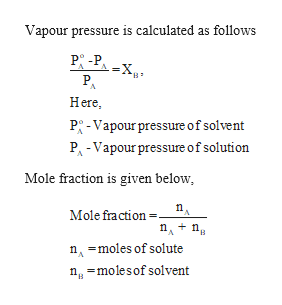The vapor pressure of ethanol (CH3CH2OH) is 54.7 mm Hg at 25 °C. What is the vapor pressure of a solution consisting of 231 g of ethanol and 0.190mol of a nonvolatile nonelectrolyte? Answer:VP(solution) =  _____mm Hg

Question

The vapor pressure of ethanol (CH3CH2OH) is 54.7 mm Hg at 25 °C. What is the vapor pressure of a solution consisting of 231 g of ethanol and 0.190mol of a nonvolatile nonelectrolyte?
VP(solution) =  _____mm Hg

Step 1

Given,

Molar mass of ethanol is 46 g...help_outlineImage TranscriptioncloseVapour pressure is calculated as follows P -P A=X. PA Here B P-Vapour pressure of solvent PA-Vapour pressure of solution Mole fraction is given below Mole fraction= n n nmoles of solute nmolesof solvent fullscreen

Want to see the full answer?

See Solution

Want to see this answer and more?

Our solutions are written by experts, many with advanced degrees, and available 24/7

See Solution
Tagged in

General Chemistry